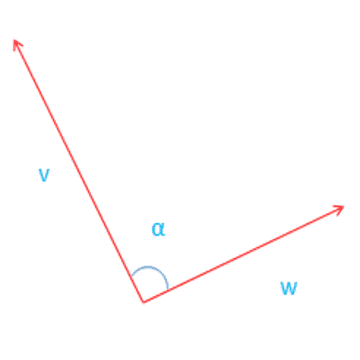# Two Dimensional Vector Angle Calculation

Using this Online Calculator, we can Calculate the Angle for two vectors v & w In 2D (Two Dimensional) vector there is only one degree of freedom for 2D rotations. If v and w are normalized so that |v|=|w|=1, then angle = inverse of cosine (v X w)
where: |v|,|w| is magnitude of v,w.Vector v: , Vector w: , Angle: Degrees

In the case of two dimension vectors there is only one degree of freedom for rotations, and we can use the dot product to find the angle between 2 vectors.
If v and w are normalized so that |v|=|w| = 1
Therefore the angle θ between 2 vectors v and w can be find as angle = arccos(|v||w|v•w)
where:
• • = dot- product
• arc cos = inverse of cosine function
• |v|= magnitude of vector v
Mostly acos returns a value between 0 and π which is 0° and 180°.